# Alias Method离散分布随机取样

## Alias-Method 原理

1. 初始概率分布: 类别数目$K=4$，以颜色表示不同的类别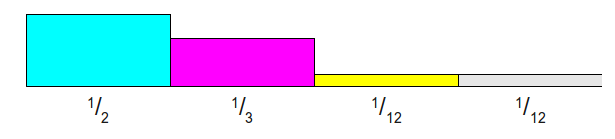2. 每个类别概率乘以K=4,使得总和为4. 这样分为两类，大于1:第一列与第二列; 小于1: 第三列与第四列。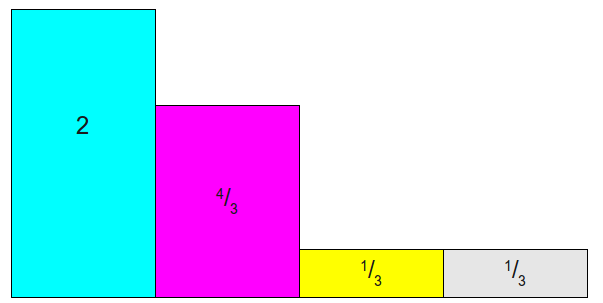3. 下面通过拼凑，使得每一列的和都为1，但是每一列中，最多只能是两种类型的拼凑，就是每一列最多两种颜色存在
• 将第一列拿出$\frac{2}{3}$给最后一列，使其变为1，如下:(棕色表示空缺)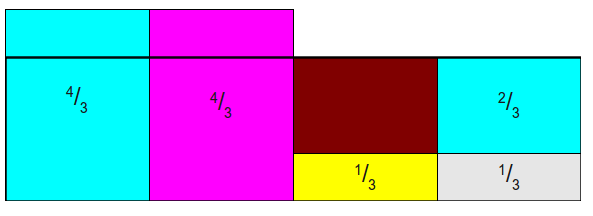• 将第一列拿出$\frac{2}{3}$给第三列，使之变为1，如下: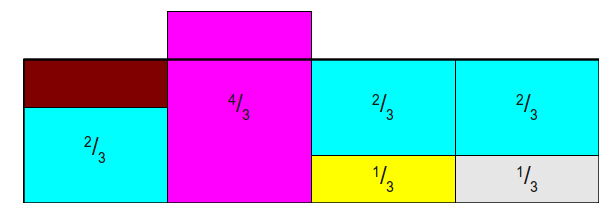• 最后一次,第二列给第一列$\frac{1}{3}$, 最后每一列都是1，且每一列最多两种类型，其中下面一层表示原类的概率，上面层表示另外一种类型的概率，如只有一种比如第二列，那么第二层就是NULL: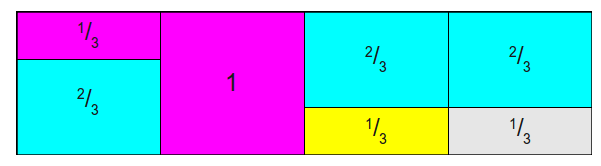4. 写出两个数组:

• Probability table $Prob$: 落在原类型的概率，每一列第一层的概率，即:$[\frac{2}{3}, 1, \frac{1}{3}, \frac{1}{3}]$
• Alias table $Alias$ : 每一列第二层的类型(颜色)，这里用下标表示: $[2, null, 1, ,1]$

用图表示如下: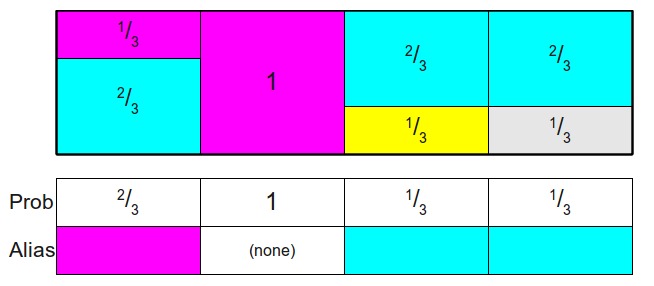到此为止，得到$Prob, Alias$ 表示初始化完成。

5. 采样过程: 随机取某一列k(即[1,4]的随机整数)，再随机产生一个[0-1]的小数c，如果$Prob[k]$ 大于 c，那么采样结果就是k，反之则为$Alias[k]$